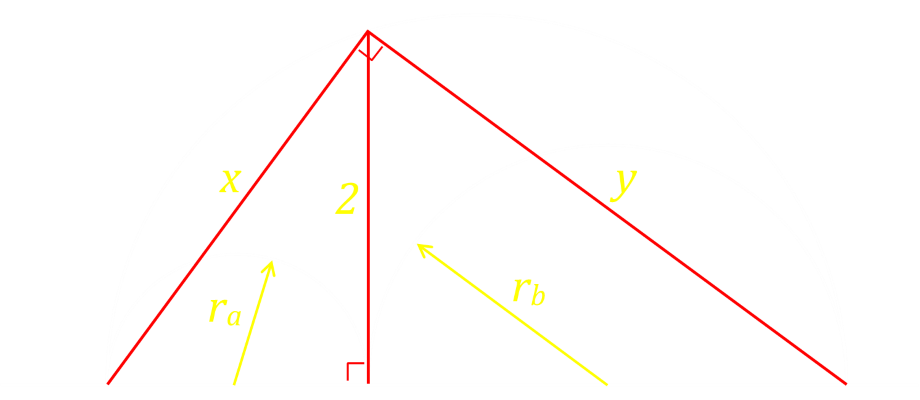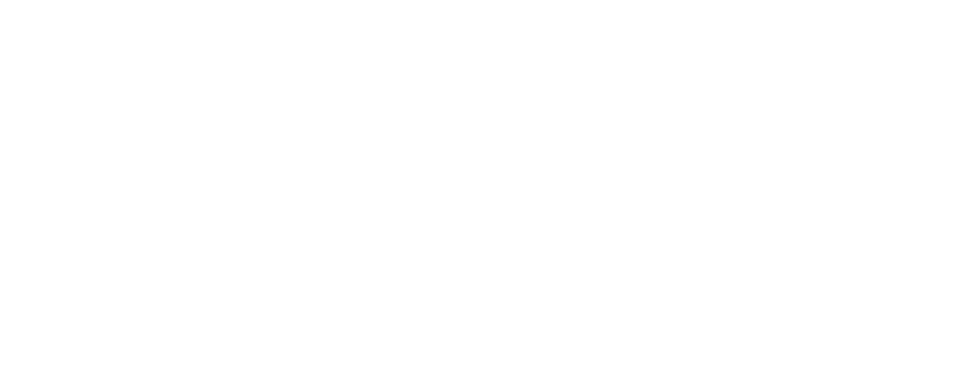# Semicircle Puzzle

The following puzzle, created by the very talented Catriona Shearer, appeared on my feed the other day.
"The red line, of length 2, is perpendicular to the bases of the three semicircles. What is the total shaded area?"This is one of those questions to which, at first glance, it appears that there is not enough information to solve. Where is the red line located? As you know, however, that question has to be solvable as is (otherwise why ask it as a puzzle?), you can deduce, that the solution has to be agnostic of the position of the red line (otherwise its position would be given).Knowing this, we can move the red line to a position that is convenient, geometrically, for us to solve; for instance right in the middle. With the line bisecting the semicircle, the red line becomes a a radius of two units.
(This is kind of a little bit cheating, and we'll investigate the generic solution below to prove this solution is agnostic of the location of the red line).
In this configuration, the large circle has radius 2, so the total area the semicircle is 2π. From this we need to subtract the two smaller semicircles, which combine to make a complete circle of radius one unit, which is just π
The area of the shaded region is, therefore, simply π units squared.

## The Napkin Ring Problem

Probably the most famous puzzle that falls into this category of appearing to not be adequately defined is The Napkin Ring Problem, created by Martin Gardner. It's the puzzle he's most proud of creating."A six inch high cylindrical hole is drilled through the center of a sphere. How much volume is left in the sphere?"

## Returning to the problem

Returning to the original problem, here is a little animation that allows you to play with the position of the vertical line. Use your mouse or finger to drag the line. The semicircles change in size to keep the vertical divider at two units tall.

## Generic Solution

Here is a diagram of the system. The radius of the two small circles are ra and rbThere are three right angle triangles in the system. Using Pythagoras for the left and right triangles (which have bases that are the diameters of the two smaller semicircles):Adding both of these we get:There is also another, larger, triangle which has a hypotenuse of the diameter of the larger circle:We now have two equations for x2+y2, which we can combine and simplify:The result we find is that there is a reciprocal arrangement between ra and rb and their product is one.
To calculate the shaded area, we start out with the area of the large semicircle, and subtract the two smaller ones.We see that this equation has the term of the product of the two smaller radii, and we can substitute this in to give the final result:We have confirmed the answer we achieved before!